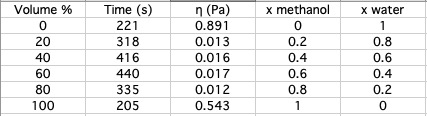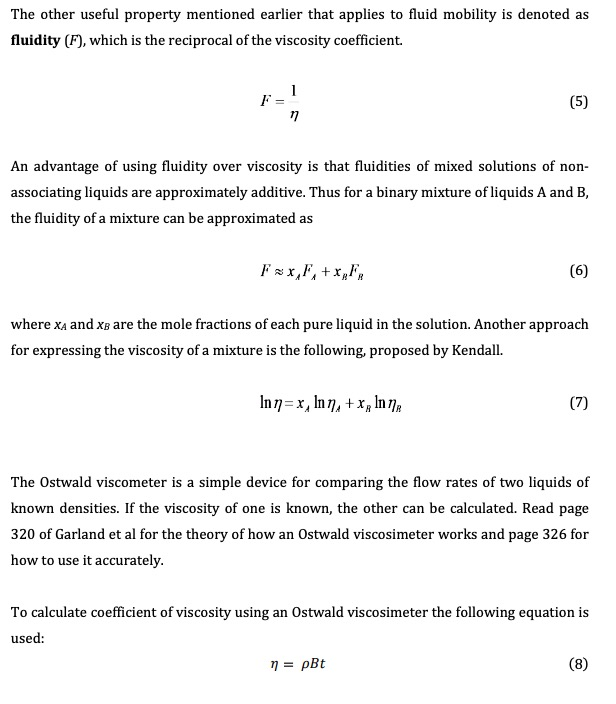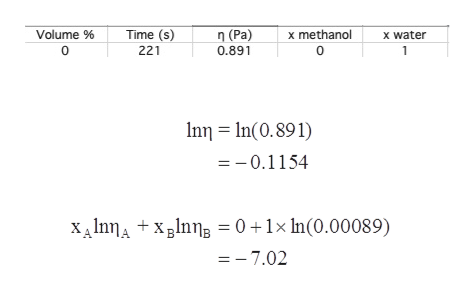# Time (s)n(Pa)Volume %x methanolx water2210.89101318200.0130.20.8404160.0160.40.6604400.0170.60.40.2803350.0120.80.5431002051 The other useful property mentioned earlier that applies to fluid mobility is denoted asfluidity (F), which is the reciprocal of the viscosity coefficientF =(5)An advantage of using fluidity over viscosity is that fluidities of mixed solutions of non-associating liquids are approximately additive. Thus for a binary mixture of liquids A and B,the fluidity of a mixture can be approximated asFx,F,+xF(6)where xA and xB are the mole fractions of each pure liquid in the solution. Another approachfor expressing the viscosity of a mixture is the following, proposed by Kendall(7)The Ostwald viscometer is a simple device for comparing the flow rates of two liquids ofknown densities. If the viscosity of one is known, the other can be calculated. Read page320 of Garland et al for the theory of how an Ostwald viscosimeter works and page 326 forhow to use it accurately.To calculate coefficient of viscosity using an Ostwald viscosimeter the following equation isused:(8)pBt

Question
15 views

Use the data and formula below to test the validity of the equation (7) for the two binary systems studied.help_outlineImage TranscriptioncloseTime (s) n(Pa) Volume % x methanol x water 221 0.891 0 1 318 20 0.013 0.2 0.8 40 416 0.016 0.4 0.6 60 440 0.017 0.6 0.4 0.2 80 335 0.012 0.8 0.543 100 205 1 fullscreenhelp_outlineImage TranscriptioncloseThe other useful property mentioned earlier that applies to fluid mobility is denoted as fluidity (F), which is the reciprocal of the viscosity coefficient F = (5) An advantage of using fluidity over viscosity is that fluidities of mixed solutions of non- associating liquids are approximately additive. Thus for a binary mixture of liquids A and B, the fluidity of a mixture can be approximated as Fx,F,+xF (6) where xA and xB are the mole fractions of each pure liquid in the solution. Another approach for expressing the viscosity of a mixture is the following, proposed by Kendall (7) The Ostwald viscometer is a simple device for comparing the flow rates of two liquids of known densities. If the viscosity of one is known, the other can be calculated. Read page 320 of Garland et al for the theory of how an Ostwald viscosimeter works and page 326 for how to use it accurately. To calculate coefficient of viscosity using an Ostwald viscosimeter the following equation is used: (8) pBt fullscreen
check_circle

Step 1

Viscosity is defined as the liquid’s resistance to flow. It is another type of bulk property.

Step 2

Assuming the coefficient of viscosity for methanol and water as 0.000594 Pa.s and 0.00089 Pa.s.

The validity of the equation represented as follows:

Step 3

Now to check the validity of equation represented abo...help_outlineImage Transcriptionclosex methanol Volume % Time (s) n(Pa) x water 221 0.891 0 1 n n(0.891) =-0.1154 XAlnnAxlnn = 0 + 1x In(0.00089) =7.02 fullscreen

### Want to see the full answer?

See Solution

#### Want to see this answer and more?

Solutions are written by subject experts who are available 24/7. Questions are typically answered within 1 hour.*

See Solution
*Response times may vary by subject and question.
Tagged in

### Chemistry